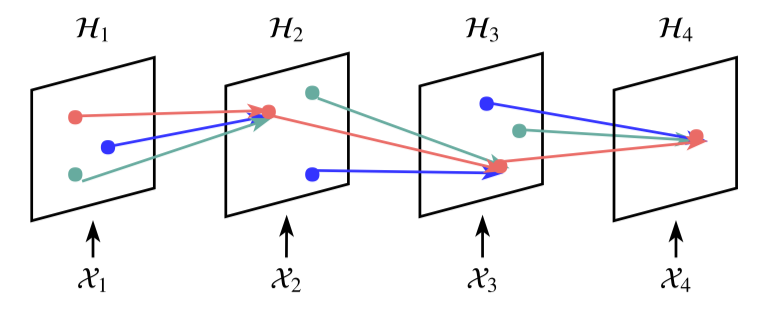# 关于 TrajGRU 的意义 #11

Closed
opened this issue May 2, 2019 · 0 comments

 最近我复现了 ConvLSTM 和 TrajGRU。TrajGRU 的参数和作者一样，和论文的结果基本相同。 其他参数一样，我只将 RNN 替换成 ConvLSTM，卷积核都是kernel 3, stride 1, padding 1。 结果很令我吃惊，我在复现 ConvLSTM 后，发现部分结果比论文 report 的结果要好，或者接近。 我用 validation set 验证，然后选出一个在 test set 测试，以上结果是 test set 上的。 按照论文的结果，TrajGRU 可以建立动态的递归连接：那么为什么会出现这样的结果呢？ 下面是的 ConvLSTM 的实现： ```from torch import nn import torch from nowcasting.config import cfg class ConvLSTM(nn.Module): def __init__(self, input_channel, num_filter, b_h_w, kernel_size, stride=1, padding=1): super().__init__() self._conv = nn.Conv2d(in_channels=input_channel + num_filter, out_channels=num_filter*4, kernel_size=kernel_size, stride=stride, padding=padding) self._batch_size, self._state_height, self._state_width = b_h_w self.Wci = torch.zeros(1, num_filter, self._state_height, self._state_width).to(cfg.GLOBAL.DEVICE) self.Wcf = torch.zeros(1, num_filter, self._state_height, self._state_width).to(cfg.GLOBAL.DEVICE) self.Wco = torch.zeros(1, num_filter, self._state_height, self._state_width).to(cfg.GLOBAL.DEVICE) self.Wci.requires_grad = True self.Wcf.requires_grad = True self.Wco.requires_grad = True self._input_channel = input_channel self._num_filter = num_filter # inputs and states should not be all none # inputs: S*B*C*H*W def forward(self, inputs=None, states=None, seq_len=cfg.HKO.BENCHMARK.IN_LEN): if states is None: c = torch.zeros((inputs.size(1), self._num_filter, self._state_height, self._state_width), dtype=torch.float).to(cfg.GLOBAL.DEVICE) h = torch.zeros((inputs.size(1), self._num_filter, self._state_height, self._state_width), dtype=torch.float).to(cfg.GLOBAL.DEVICE) else: h, c = states outputs = [] for index in range(seq_len): # initial inputs if inputs is None: x = torch.zeros((h.size(0), self._input_channel, self._state_height, self._state_width), dtype=torch.float).to(cfg.GLOBAL.DEVICE) else: x = inputs[index, ...] cat_x = torch.cat([x, h], dim=1) conv_x = self._conv(cat_x) i, f, tmp_c, o = torch.chunk(conv_x, 4, dim=1) i = torch.sigmoid(i+self.Wci*c) f = torch.sigmoid(f+self.Wcf*c) c = f*c + i*torch.tanh(tmp_c) o = torch.sigmoid(o+self.Wco*c) h = o*torch.tanh(c) outputs.append(h) return torch.stack(outputs), (h, c)``` 我把八个卷积操作合并了。 TrajGRU 的实现我是根据作者的代码实现的，逻辑类似。在其他参数相似的情况下，inference 的速度 TrajGRU 要比 ConvLSTM 差很多，分别是 0.0320 样本/s 和 0.4120 样本/s(我认为 subnetwork 的影响很小，毕竟这个网络很简单)。 所以在速度相差这么大、性能差不多的情况下，如果实际应用，为什么要考虑 TrajGRU 呢？